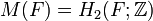# Schur multiplier of free group is trivial

1. For a free group$F$, the commutator map homomorphism from the exterior square$F \wedge F$ to the derived subgroup$[F,F]$ is an isomorphism.
2. For a free group$F$, the Schur multiplier$M(F) = H_2(F;\mathbb{Z})$ is the trivial group.